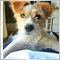# How the "and" & "or" operators work, when both combined in an if statement.194

Hi there everyone, this is just confusing me, and I need to get my ordering right.

if( A < B  &&  C < D  ||  E < F ).

In that statement, does it mean:

if ( A < B  &&  C < D ) "or" (A < B  &&  E < F )

or does it mean:

if ( A < B  &&  C < D ) "or" ( E < F ).

In other words, does the "or" operator effectively start the if statement again (in the second example), or does it simply mean either one of the statements either side of the "or" operator itself (as in the first example).

Its kind of hard to explain.3201

it means:

if ( A < B  &&  C < D ) "or" ( E < F ).194

Thanks17374

qjol:
it means:

if ( A < B  &&  C < D ) "or" ( E < F ).

No it doesn't.

It actually means . . .

`if( A < B  &&  ( C < D  ||  E < F ) )`

. . . due to the Precedence rules  the OR ( || )  has a higher precedence than the AND ( && )

For clarity use braces ( )  to make the code do what you want it to do . . .  then you don't have to figure out what the Precedence rules mean for your code.[Deleted]

Its always a good practice to separate different sets of comparisons with brackets. See below examples.

```if( A < B  &&  (C < D  ||  E < F) ) // If A less than B(compulsary condition) then either C is less than D(option 1) or E is less than F(option 2)

if( (A < B  && C < D)  ||  E < F ) // If either A is less than B and also C less than D(Option 1) or alternatively E is less than F(option 2) ```24704

Or use boolean variables with good naming and make your code self documenting:

With BooleansPlain
```bool isCondition1 = A < B,
isCondition2 = C < D || E < F;
if (isCondition1 && isCondition2) ..```
`if( A < B  &&  (C < D  ||  E < F) )`

```bool isCondition1 = A < B && C < D,
isCondition2 = E < F;
if (isCondition1 || isCondition2) ..```

`if( (A < B  && C < D)  ||  E < F )`18

Been reading and thanks for all your pointers. I cant seem to figure out a more simpler way of writing this :

```//CODE EXAMPLE 1 :
else if ((alertswitch == -2 ||alertswitch == -4 ||alertswitch == -6 ||alertswitch == -51 ||alertswitch == 81)
&& CurrentPrice > PbarHigh
&& (alertswitch != 70  && alertswitch != 0 ))
{Check=70;}

//CODE EXAMPLE 2 :
else if ((alertswitch == -2 ||-4 ||-6 ||-51 ||81)              //I prefer example 2 but it's not working.
&& CurrentPrice > PbarHigh
&& (alertswitch != 70  && 0 ))
{Check=70;}```